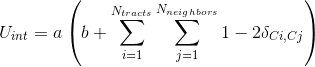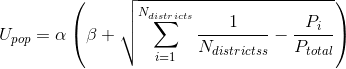# Toward a Physically-Justified Redistricting Methodology

Districts:
Population Energy = xxx
Interaction Energy = xxx
Total Energy = xxx
Percent Acceptance: 0
Population Disparity: xxx

Temperature = xxx

## Introduction

This is a Javascript program I wrote to sample redistricting in North Carolina with the Metropolis Monte Carlo method. Based on Monte Carlo simulations of phase equilibrium, I wondered what would happen if a model NC followed quasi-physical rules for apportioning Congressional districts. The model is an Ising-type model on a lattice of census tracts, according to the 2010 US census. The code isn't perfect, but it should let you play around with the basic idea of NCMC (North Carolina Monte Carlo). See what minimum energy or population disparity you can get! (Maybe try an advanced sampling technique like simulated annealing??)

## Methods

The lattice is a graph of census tracts, where each vertex is a tract and they share an edge if the census tract boundaries share a point in common. Basically if the census tracts touch, they're neighbors. For each move, the code picks a random census tract on the border between districts and attempts to flip it to the adjacent district. If the energy change is favorable (negative), the move takes place. If the move is unfavorable (positive energy change), there is a probability of acceptance based on the energy difference and temperature. Higher temperature means higher probability of acceptance and higher-energy configurations, which can let the system "jump over" energy barriers.
The energy is composed of two terms; first is the Ising-type interaction energywhere a and b are arbitrary constants to scale and shift the energy distribution, the delta is the Kronecker delta function, and Ci and Cj are the "colors" (district numbers) of tract i and j, respectively. The interaction energy is summed over all neighbors of each census tract. In this way, tracts with neighbors in the same district have a favorable (negative energy) interaction, while otherwise the interaction is unfavorable (positive energy) if the tract's neighbors are in a different district.
The second energy term is the population energywhere α and β are again arbitrary constants to scale and shift the population energy distribution and Ptotal and Pi are the total population and the population of district i, respectively. This is kind of like the standard deviation of the set of district populations and imposes an energy penalty (positive energy) if the district populations are too uneven.